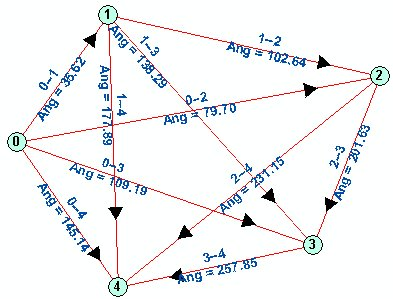Go to ET GeoWizards 12.x User Guide

Connect Points

Go to ToolBox Implementation

Connects with lines each point from a point dataset to every other point from the same dataset that is closer to the point than the user defined cut off distance.

• Assigns the IDs of the From and To Points to each line..
• Calculates the length and angle of the connector lines.

Inputs:

• A Point feature class
• Generalization tolerance - should be smaller than 20% of the smaller side of the extent envelope of the input dataset.
• Data field - if specified the output feature class will have a field in which the value for each resulting point will be the sum of the values of the points pertaining to this cluster.

Outputs:

• New point feature class. Fields:
• [ET_From] - the ID of the start point of the line
• [ET_To] - the ID of the end point of the line
• [ET_Length] - the length of the line
• [ET_Angle] - the angle of the line

Notes:

• If no Cutoff distance tolerance is specified each point will be connected to all other points
• Important: The number of connector lines created if no cutoff distance is used can be calculated using the formulae N = n x (n-1)/2 (N - number of lines, n - number of input points). For example 1,000 points will create 499,500 lines, 10,000 points will create 49,995,000 lines, which is not a dataset you want to handle.

Example:Each set of points will be connected only once. Point 0 connects to Points 1, 2, 3, 4 Point 1 connects to Points 2, 3, 4 (it already has been connected to Point 0) Point 2 connects to Points 3, 4 (it already has been connected to Points 0 and 1) ...... The angle is calculated in North Azimuth directionCommand line syntax

ET_GPConnectPoints<input_dataset> <out_feature class> {Cutoff_distance}

Parameters

Expression Explanation
<input_dataset> A  Point feature class or feature layer
<out_feature_ class> A String - the full name of the output feature class (A feature class with the same full name should not exist)
{Cutoff_distance} A Double representing the Generalization tolerance.

Scripting syntax

ET_GPThinPoints (input_dataset,  out_feature_ class, Cutoff_distance)

See the explanations above:
<> - required parameter
{} - optional parameter